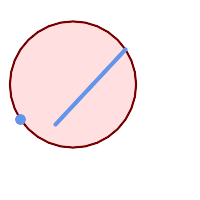## Name

ST_MinimumBoundingCircle — Returns the smallest circle polygon that contains a geometry.

## Synopsis

`geometry ST_MinimumBoundingCircle(`geometry geomA, integer num_segs_per_qt_circ=48`)`;

## Description

Returns the smallest circle polygon that contains a geometry.The bounding circle is approximated by a polygon with a default of 48 segments per quarter circle. Because the polygon is an approximation of the minimum bounding circle, some points in the input geometry may not be contained within the polygon. The approximation can be improved by increasing the number of segments. For applications where an approximation is not suitable ST_MinimumBoundingRadius may be used.

Use with ST_Collect to get the minimum bounding circle of a set of geometries.

To compute two points lying on the mimimum circle (the "maximum diameter") use ST_LongestLine.

The ratio of the area of a polygon divided by the area of its Minimum Bounding Circle is referred to as the Reock compactness score.

Performed by the GEOS module.

Availability: 1.4.0

## Examples

```SELECT d.disease_type,
ST_MinimumBoundingCircle(ST_Collect(d.geom)) As geom
FROM disease_obs As d
GROUP BY d.disease_type;
```Minimum bounding circle of a point and linestring. Using 8 segs to approximate a quarter circle

```SELECT ST_AsText(ST_MinimumBoundingCircle(
ST_Collect(
ST_GeomFromText('LINESTRING(55 75,125 150)'),
ST_Point(20, 80)), 8
)) As wktmbc;
wktmbc
-----------
POLYGON((135.59714732062 115,134.384753327498 102.690357210921,130.79416296937 90.8537670908995,124.963360620072 79.9451031602111,117.116420743937 70.3835792560632,107.554896839789 62.5366393799277,96.6462329091006 56.70583703063,84.8096427890789 53.115246672502,72.5000000000001 51.9028526793802,60.1903572109213 53.1152466725019,48.3537670908996 56.7058370306299,37.4451031602112 62.5366393799276,27.8835792560632 70.383579256063,20.0366393799278 79.9451031602109,14.20583703063 90.8537670908993,10.615246672502 102.690357210921,9.40285267938019 115,10.6152466725019 127.309642789079,14.2058370306299 139.1462329091,20.0366393799275 150.054896839789,27.883579256063 159.616420743937,
37.4451031602108 167.463360620072,48.3537670908992 173.29416296937,60.190357210921 176.884753327498,
72.4999999999998 178.09714732062,84.8096427890786 176.884753327498,96.6462329091003 173.29416296937,107.554896839789 167.463360620072,
117.116420743937 159.616420743937,124.963360620072 150.054896839789,130.79416296937 139.146232909101,134.384753327498 127.309642789079,135.59714732062 115))
```